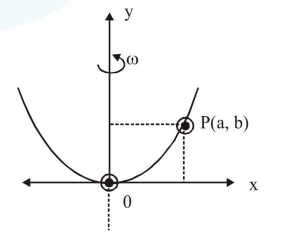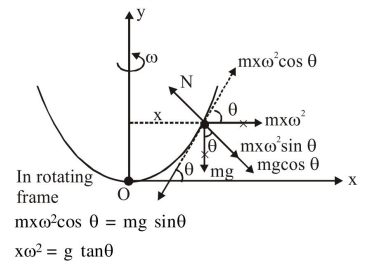# Solve this following`
Question:

A bead of mass $m$ stays at point $P(a, b)$ on a wire bent in the shape of a parabola $\mathrm{y}=4 \mathrm{Cx}^{2}$ and rotating with angular speed $\omega$ (see figure). The value of $\omega$ is (neglect friction):1. $\sqrt{\frac{2 \mathrm{gC}}{\mathrm{ab}}}$

2. $2 \sqrt{2 \mathrm{gC}}$

3. $\sqrt{\frac{2 g}{\mathrm{C}}}$

4. $2 \sqrt{\mathrm{gC}}$

Correct Option: , 2

Solution:$x \omega^{2}=g \cdot \frac{d y}{d x}$

$x \omega^{2}=g .(8 c x)$

$\omega^{2}=8 \mathrm{gc}$

$\omega=2 \sqrt{2 \mathrm{gc}}$